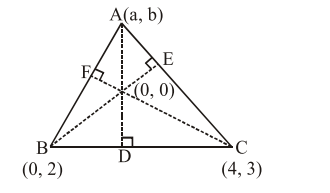Deepak Scored 45->99%ile with Bounce Back Crack Course. You can do it too!

# Two vertices of a triangle are

Question:

Two vertices of a triangle are $(0,2)$ and $(4,3)$. If its orthocentre is at the origin, then its third vertex lies in which quadrant ?

1. Fourth

2. Second

3. Third

4. First

Correct Option: , 2

Solution:

$\mathrm{m}_{\mathrm{BD}} \times \mathrm{m}_{\mathrm{AD}}=-1 \Rightarrow\left(\frac{3-2}{4-0}\right) \times\left(\frac{\mathrm{b}-0}{\mathrm{a}-0}\right)=-1$

$\Rightarrow b+4 a=0$ ...............(I)$\mathrm{m}_{\mathrm{AB}} \times \mathrm{m}_{\mathrm{CF}}=-1 \Rightarrow\left(\frac{(\mathrm{b}-2)}{\mathrm{a}-0}\right) \times\left(\frac{3}{4}\right)=-1$

$\Rightarrow 3 b-6=-4 a \Rightarrow 4 a+3 b=6 \quad \ldots \ldots$ (ii)

From (i) and (ii)

$a=\frac{-3}{4}, b=3$

$\therefore \quad$ II ${ }^{\text {nd }}$ quadrant.

Option (2)